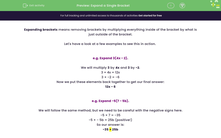# Expand a Single Bracket

In this worksheet, students will expand one set of brackets in calculations and problems (i.e. multiply to remove them), expressing answers in their simplest forms.Key stage:  KS 4

Year:  GCSE

GCSE Subjects:   Maths

GCSE Boards:   Pearson Edexcel, OCR, Eduqas, AQA,

Curriculum topic:   Algebra

Curriculum subtopic:   Notation, Vocabulary and Manipulation Algebraic Expressions

Difficulty level:#### Worksheet Overview

Expanding brackets means removing brackets by multiplying everything inside of the bracket by what is just outside of the bracket.

Let's have a look at a few examples to see this in action.

e.g. Expand 3(4x − 2).

We will multiply 3 by 4x and 3 by −2.

3 × 4x = 12x

3 × −2 = −6

Now we put these elements back together to get our final answer:

12x − 6

e.g. Expand −5(7 − 5b).

We will follow the same method, but we need to be careful with the negative signs here.

−5 × 7 = −35

−5 × − 5b = 25b (positive!)

−35 + 25b

Remember:

When we multiply a negative by a positive number, our answer is negative.

When we multiply two negative numbers, our answer will be positive.

In this activity, we will expand one set of brackets in calculations and problems, expressing our answers in their simplest forms.

### What is EdPlace?

We're your National Curriculum aligned online education content provider helping each child succeed in English, maths and science from year 1 to GCSE. With an EdPlace account you’ll be able to track and measure progress, helping each child achieve their best. We build confidence and attainment by personalising each child’s learning at a level that suits them.

Get started